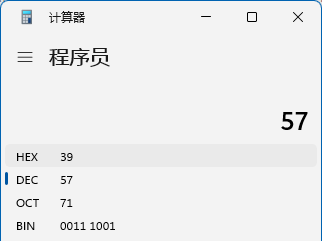• Java 教程
• Java 简介
• Java 基础
• Java 运算符
• Java 表达式
• Java 流程控制
• Java 数组
• Java 面向对象
• Java 集合类
• Java 异常处理
• Java 泛型
• Java 内置工具
• 参考手册

# Java 位运算符Java 中位运算符用于对整型数据的二进制位进行运算，具体包括如下：

• ~    按位取反，即 0 取反为 1，1 取反为 0

• &    按位与，即 1 和 1 进行与运算为 1，其它均为 0

• |    按位或，即 0 和 0 进行或运算为 0，其它均为 0

• ^    按位异或，即 1 和 0，或者 0 和 1 进行或运算为 1，其它均为 0

 a b ~a a & b a | b a ^ b 1 1 0 1 1 0 1 0 0 0 1 1 0 1 1 0 1 1 0 0 1 0 0 0

## 示例

```import java.util.Arrays;

public class Demo {

public static void main(String[] args) {
int a = 20, b = 35;
// 注意 Integer.toBinaryString() 方法是 Integer 类中的静态工具方法
// 用来将数字使用二进制字符串显示
System.out.println("  a=" + format(Integer.toBinaryString(a)));
System.out.println("  b=" + format(Integer.toBinaryString(b)));
// 按位取反
System.out.println(" ~a=" + format(Integer.toBinaryString(~a)));
// 按位与
System.out.println("a&b=" + format(Integer.toBinaryString(a & b)));
// 按位或
System.out.println("a|b=" + format(Integer.toBinaryString(a | b)));
// 按位异或
System.out.println("a^b=" + format(Integer.toBinaryString(a ^ b)));
}

/**
* 格式化字符串，保持字符串长度为32，如果长度不够，左边填充字符0
* @param str 带格式化字符串
* @return
*/
private static String format(String str) {
if(str.length() > 32) {
return str;
}

// 实现左端用字符0补齐
char[] chars = new char[32 - str.length()];
Arrays.fill(chars, '0');
String tmpStr = String.copyValueOf(chars) + str;

// 这里使用了 Java 的 StringBuilder，后续会介绍
StringBuilder buffer = new StringBuilder();
for(int i = 0; i < tmpStr.length(); i++) {
if(i > 0 && i % 8 == 0) {
buffer.append(" ");
}
buffer.append(tmpStr.charAt(i));
}

return buffer.toString();
}

}```

```  a=00000000 00000000 00000000 00010100
b=00000000 00000000 00000000 00100011
~a=11111111 11111111 11111111 11101011
a&b=00000000 00000000 00000000 00000000
a|b=00000000 00000000 00000000 00110111
a^b=00000000 00000000 00000000 00110111```